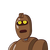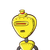# Perimeter of a rectangle is 24 meters. If length of a rectangle is 2 meters more than its breadth, then find the breadth of a rect

Perimeter of a rectangle is 24 meters. If length of a rectangle is 2 meters more than its breadth, then find the breadth of a rectangle.

### 2 thoughts on “Perimeter of a rectangle is 24 meters. If length of a rectangle is 2 meters more than its breadth, then find the breadth of a rect”

1.Diagram :

$$\setlength{\unitlength}{1cm}\begin{picture}(0,0)\thicklines\multiput(0,0)(5,0){2}{\line(0,1){3}}\multiput(0,0)(0,3){2}{\line(1,0){5}}\put(0.03,0.02){\framebox(0.25,0.25)}\put(0.03,2.75){\framebox(0.25,0.25)}\put(4.74,2.75){\framebox(0.25,0.25)}\put(4.74,0.02){\framebox(0.25,0.25)}\multiput(2.1,-0.7)(0,4.2){2}{\sf\large x+2\: m}\multiput(-1.4,1.4)(6.8,0){2}{\sf\large x m}\put(-0.5,-0.4){\bf A}\put(-0.5,3.2){\bf D}\put(5.3,-0.4){\bf B}\put(5.3,3.2){\bf C}\end{picture}$$

❍ Let’s Consider the Breadth of Rectangle be x m

Then ,

Given that ,

• Length of a rectangle is 2 meters more than its breadth.

Therefore,

• Length of Rectangle is x + 2 m

$$\dag\frak{\underline {Perimeter \:of\:Rectangle \::}}\\$$

$$\star\boxed {\sf{\pink{ Perimeter_{(Rectangle)} = 2 ( l + b) \:units }}}\\\\$$

Where ,

• l is the Length of Rectangle and b is the Breadth of Rectangle.

⠀⠀⠀⠀⠀⠀$$\underline {\bf{\star\:Now \: By \: Substituting \: the \: known \: Values \::}}\\$$

$$:\implies \sf { 24 = 2(x + x + 2) }\\\\ :\implies \sf { \cancel {\dfrac{24}{2}} = x + x + 2 }\\\\ :\implies \sf { 12 = x + x + 2 }\\\\ :\implies \sf { 12 = 2x + 2 }\\\\ :\implies \sf { 12-2 = 2x }\\\\ :\implies \sf { 10 = 2x }\\\\ :\implies \sf { \cancel {\dfrac{10}{2}} = x }\\\\\underline {\boxed{\pink{ \mathrm { x = 5\: m}}}}\:\bf{\bigstar}\\$$

Therefore,

• Length of Rectangle is (x +2) = 5 + 2 = 7 m
• Breadth of Rectangle is x = 5 m

Therefore,

⠀⠀⠀⠀⠀$$\therefore {\underline{ \mathrm { Hence,\: Breadth \:of\:Rectangle \:is\:\bf{5\: m}}}}\\$$

⠀⠀⠀⠀⠀━━━━━━━━━━━━━━━━━━━⠀

2.